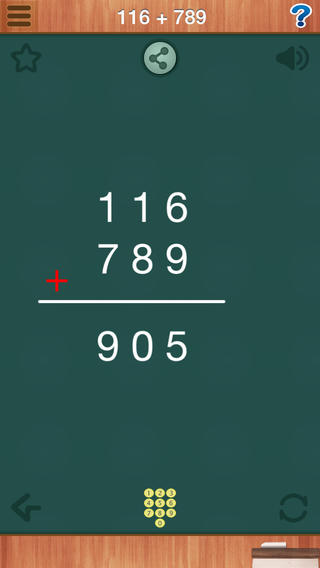Maths Animations (Ages 6-14 Years): Includes numbers, decimals, fractions, addition, subtraction, muGreat way to "see" how math works! --by Greg Garner

Awesomely Effective!!! An absolutely amazing and brilliant way to visually demonstrate basic math principles! Thank you! -- by iPad Dad3

Maths Animations : mathematics animations. The animations provide short, sharp, visual explanations of mathematical concepts.

Who is it for?

It is for everyone interested in mathematics. It is principally designed for upper primary and lower secondary teachers to use in the classroom, but students interested in deepening their understanding of core concepts will also find the site useful.

List of animations:3. Subtraction

4. Subtraction Table

5. Multiplication

6. Multiplication Table

7. Division8. Division Table

9. 123 = ?

10. 12 + 45

11. 123 + 456

12. 45 - 12

13. 456 - 123

14. 12 × 315. 12 × 45

16. 123 × 56

17. 456 ÷ 3

18. 456 ÷ 12

19. a + b = b + a

20. a × b = b × a

21. (a+b) × c = a×c + b×c22. Perimeter of a Square

23. Perimeter of a Rectangle

24. What is Area?

25. Area of a Square

26. Area of a Rectangle

27. Area of a Parallelogram

28. Area of a Triangle29. Area of a Trapezoid

30. Angle sum of a Triangle

31. Definition of a circle

32. Radius × 2 = Diameter

33. What is π ?

34. Perimeter of a circle

35. Area of a circle36. What is Fraction?

38. Subtracting Fractions

39. Multiplying Fractions

40. Dividing Fractions

41. Fraction × n

42. Fraction ÷ n43. 1.23 + 0.56

44. 1.23 - 0.56

45. 1.2 × 0.5

46. 1.2 × 4.5

47. 1.23 × 0.56

48. 4.56 ÷ 2

49. 4.56 ÷ 1.250. X + 3 = 12

51. 2X = 8

52. 2X + 3 = 11免費玩Maths Animations (Ages 6-14 Years): Includes numbers, decimals, fractions, addition, subtraction, multiplication, division, geometry(Perimeter and Area of parallelograms, triangles, trapeziums), measurement, algebra. Primary & Secondary Mathematics. APP玩免費

Maths Animations (Ages 6-14 Years): Includes numbers, decimals, fractions, addition, subtraction, multiplication, division, geometry(Perimeter and Area of parallelograms, triangles, trapeziums), measurement, algebra. Primary & Secondary Mathematics. APP LOGOMaths Animations (Ages 6-14 Years): Includes numbers, decimals, fractions, addition, subtraction, multiplication, division, geometry(Perimeter and Area of parallelograms, triangles, trapeziums), measurement, algebra. Primary & Secondary Mathematics. APP QRCodeApp Store
6.1
App下載
NT\$ 30
2014-09-112014-09-29